Second law
Question

# Three blocks with masses m, 2m and 3m are connected by strings, as shown in the figure. After an upward force F is applied on block m, the masses move upward at constant speed $\upsilon$. What is the net force on the block of mass 2m? (g is the acceleration due to gravity)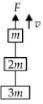Moderate
Solution

## Let ${T}_{1}$ be tension in string connecting m and 2m and ${T}_{2}$ be tension in string connecting 2m and 3m.            Let a be common acceleration of the system.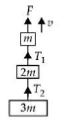$\therefore \text{\hspace{0.17em}\hspace{0.17em}}a=\frac{F-\left(m+2m+3m\right)g}{m+2m+3m}=\frac{F-6m}{6m}$As the system moves with constant speed, therefore, a = 0$\therefore \text{\hspace{0.17em}\hspace{0.17em}\hspace{0.17em}}F-6mg=0\text{\hspace{0.17em}\hspace{0.17em}}or\text{\hspace{0.17em}\hspace{0.17em}}F=6mg$The free body diagram of block m is as shown in the figure.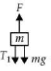The equation of motion of block of mass m isThe free body diagram of block of mass 2m is as shown in the figure.The equation of motion of block of mass 2m isThe free body diagram of block of mass3m is as shown in the figure.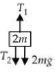The equation of motion of block of mass 3m is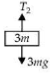Net force on the block of mass 2m isAlternate solutionAs all blocks are moving with constant speed, therefore, acceleration is zero. So net force on each block is zero.

Get Instant Solutions